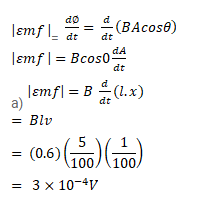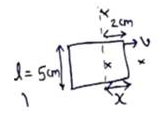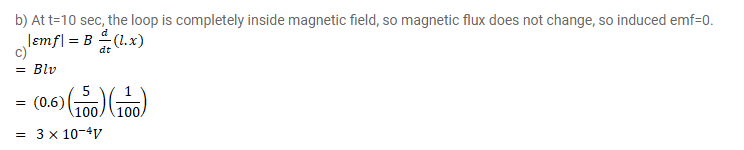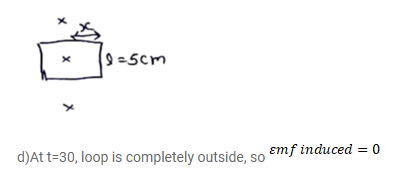# Figure shows a square loop of

Question:

Figure shows a square loop of $5 \mathrm{~cm}$ being moved towards right at a constant speed of $1 \mathrm{~cm} / \mathrm{s}$. The front edge enters the 20 $\mathrm{cm}$ wide magnetic field at $t=0$. Find the emf induced in the loop at

(a) $t=2 s$,

(b) $t=10 s$,

(c) $t=22 s$ and

(d) $t=30 s$.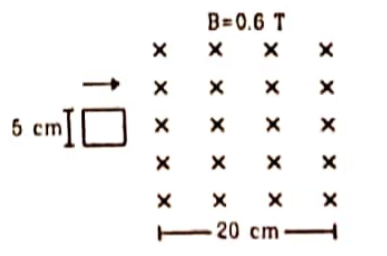Solution: